×#### Thank you for registering.

One of our academic counsellors will contact you within 1 working day.

Click to Chat

1800-1023-196

+91-120-4616500

CART 0

• 0

MY CART (5)

Use Coupon: CART20 and get 20% off on all online Study Material

ITEM
DETAILS
MRP
DISCOUNT
FINAL PRICE
Total Price: Rs.

There are no items in this cart.
Continue Shopping• Complete JEE Main/Advanced Course and Test Series
• OFFERED PRICE: Rs. 15,900
• View Details

```Revision Notes on Fractions

Fraction

A Fraction is a part of whole. The ‘whole’ here could be an object or the group of objects. But all the parts of the whole must be equal.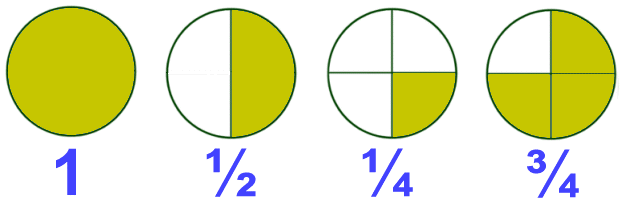The first one is the whole i.e. a complete circle.

In the second circle, if we divide the circle into two equal parts then the shaded portion is the half i.e. ½ of the circle.

In the third circle, if we divide the circle into four equal parts and shade only one part then the shaded part is the one fourth i.e. ¼ of the whole circle.

In the fourth circle, if we divide the circle into four equal parts and shade three parts then the shaded part is the three fourth i.e. ¾ of the whole circle.

Numerator and DenominatorThe upper part of the fraction is called Numerator. It tells the number of parts we have.

The lower part of the fraction is called Denominator. It tells the total parts in a whole.

Representation of fraction on Number line

Example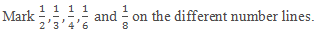Solution

Draw a number line.

We know that ½ is less than 1 and greater than 0, so we have to divide the gap between two equal parts and then mark the middle point as ½.

As the denominator is the whole and the numerator is the part, so we have to divide the gap between 0 and 1 in the number of parts as the denominator is given.

For 1/3, divide into 3 equal parts.

For ¼, divide into 4 equal parts and so on.Proper Fractions

If the numerator is less than the denominator then it is called proper fraction. If we represent a proper fraction on the number line than it will always lie between 0 and 1.

Examples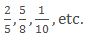Improper fractions and Mixed fractions

When the numerator is greater than the denominator then it is called Improper fraction.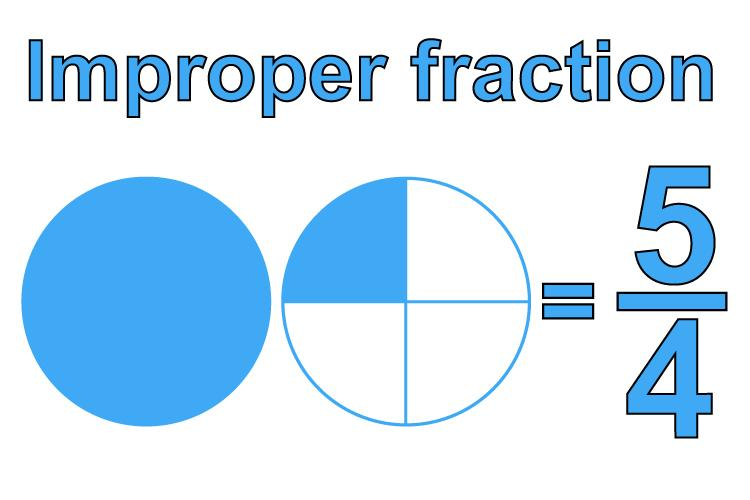The above fraction is made by adding one whole part and one-fourth part.The fraction made by the combination of whole and a part is called Mixed fraction.

Convert Mixed fraction into Improper fraction

A mixed fraction is in the form ofWe can convert it in the form of an improper fraction by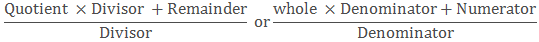Example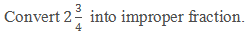SolutionEquivalent Fractions

Equivalent fractions are those fractions which represent the same part of a whole.All the above images are different but equivalent fractions as they represent the same i.e. half part of a whole circle.

Finding equivalent fractions

Multiplying the same number

If we multiply the numerator and denominator of any fraction with the same number then we will get the equivalent fraction. There could be more than one equivalent fractions of one fraction.

Example

Find three equivalent fraction of ½.

SolutionDividing the same number

If we divide the numerator and denominator of any fraction with the same number then we will get the equivalent fraction.

Example

Find the equivalent fraction of 18/27 with denominator 9.

Solution

To get the denominator 9 we need to divide it by 3.

So to find the equivalent fraction we need to divide the fraction by 3.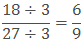Hence the equivalent fraction with denominator 9 is 6/9.

The simplest form of a Fraction

If the numerator and denominator do not have any other common factor than 1 then it is said to be the simplest or lowest form of that fraction.

Example-To find the equivalent fraction which is the simplest form we have to find the HCF of numerator and denominator and then divide them both by that HCF.

Example

Reduce the fraction 18/27 in the simplest form.

Solution

HCF of 18 and 27 is 9.

Hence,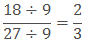2/3 is the lowest form of 18/27.

Like Fractions and Unlike Fractions

Fractions which have same denominators are known as Like fractions.

ExampleFractions which have different denominators are known as unlike fractions.

Example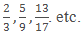Comparing fractions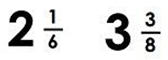If we have to compare the above two fractions then it is easy as the first one is less than 3 and the second one is greater than 3. So we can clearly say thatBut sometimes it is not easy to compare it so easily. So we need some accurate procedure.

Comparing like fractions

Like fractions are the fractions with the same denominator so we have to compare them with the numerator only. The fraction with greater numerator is greater.In the above example, both are divided into 8 equal parts, so the fraction with seven shaded part is greater than the 5 shaded parts.

Comparing unlike fractions

The fractions with different denominators are unlike fractions.

Unlike fraction with the same numerator

If we have to compare the fractions with different denominator but same numerator, we have to compare with the denominator only.

In that case, the fraction with the small denominator is greater than the other.

ExampleHere the numerator is same i.e.3 so we will compare with the denominator.

The fraction with small denominator i.e. ¾ is greater than the fraction with the large denominator i.e. 3/8.

Unlike fraction with different numerators

If the numerator and denominator both are different then we have to make the denominator same by finding the equivalent fraction of both the fractions then compare the fractions as like fractions.

To find the equivalent fraction of both the fractions with the same denominator, we have to take the LCM of the denominator.

Example

Compare 6/7 and 3/5.

Solution

The product of 7 and 5 is 35.

So we will find the equivalent fraction of both the fractions with the denominator 35.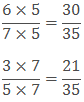Now we can compare them as like fractions.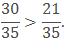In case of like fractions, the denominator is same so we can add them easily.

Leave the common denominator same. (Don’t add the denominator).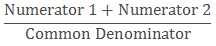Example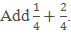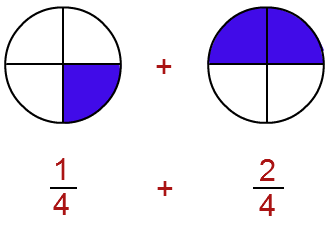Solution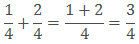Subtracting like fractions

Steps to subtract the like fractions-

Subtract the small numerator from the bigger one.

Leave the common denominator same.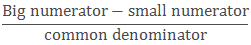ExampleSolution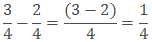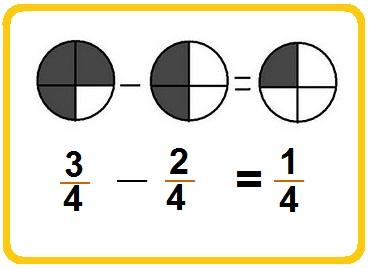If we have to add the unlike fractions, first we have to find the equivalent fraction of the given fractions with the same denominator then add them.

Take the LCM of the denominator of the given fractions.

Find the equivalent fractions of both fractions with LCM as the denominator.

Add them as the like fractions.

Example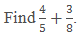Solution

Take the LCM of 5 and 8, which is 40.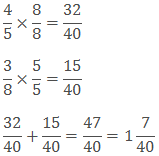Subtracting unlike fractions

Steps to subtract unlike fractions-

Take the LCM of the denominator of the given fractions.

Find the equivalent fractions of both fractions with LCM as the denominator.

Subtract them as the like fractions.

ExampleSolution

LCM of 4 and 5 is 20.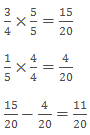Remark: To add or subtract the mixed fractions, simply convert them in the improper fraction then add and subtract them directly
```### Course Features

• 728 Video Lectures
• Revision Notes
• Previous Year Papers
• Mind Map
• Study Planner
• NCERT Solutions
• Discussion Forum
• Test paper with Video Solution